Open in App
Not now

# Data Preprocessing, Analysis, and Visualization for building a Machine learning model

• Difficulty Level : Basic
• Last Updated : 27 Oct, 2022

In this article, we are going to see the concept of Data Preprocessing, Analysis, and Visualization for building a Machine learning model. Business owners and organizations use Machine Learning models to predict their Business growth. But before applying machine learning models, the dataset needs to be preprocessed.

So, let’s import the data and start exploring it.

## Importing Libraries and Dataset

We will be using these libraries :

• Pandas library is used for data analysis.
• Numpy library is used for complex mathematical operations.
• Scikit-learn for model training and score evaluation.

## Python3

 `import` `pandas as pd``import` `numpy as np``from` `sklearn.preprocessing ``import` `LabelEncoder``from` `sklearn.preprocessing ``import` `OneHotEncoder``from` `sklearn.model_selection ``import` `train_test_split``from` `sklearn.preprocessing ``import` `StandardScaler`` ` `dataset ``=` `pd.read_csv(``'Churn_Modelling.csv'``)`

Now let us observe the dataset.

## Python3

 `dataset.head()`

Output :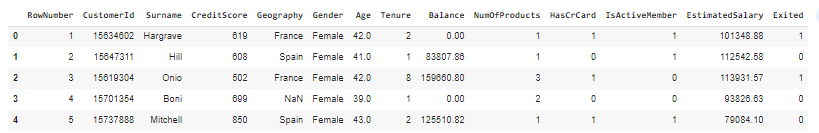Info() function retrieves the information about the dataset such as data type, number of rows and columns, etc.

## Python3

 `dataset.info()`

Output :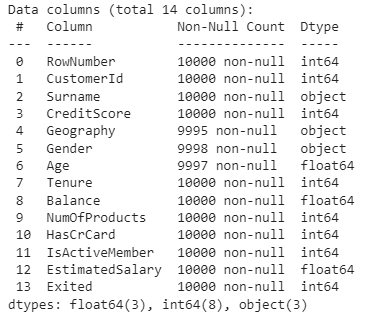## Exploratory data analysis and visualization

To find out the correlation between the features, Let’s make the heatmap

## Python3

 `plt.figure(figsize``=``(``12``,``6``))`` ` `sns.heatmap(dataset.corr(),``            ``cmap``=``'BrBG'``,``            ``fmt``=``'.2f'``,``            ``linewidths``=``2``,``            ``annot``=``True``)`

Output :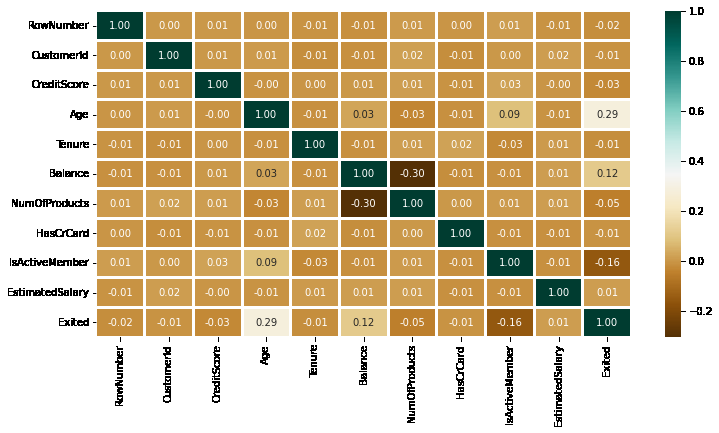Now we can also explore the distribution of CreditScore, Age, Balance, ExtimatedSalary using displot.

## Python3

 `lis ``=` `[``'CreditScore'``, ``'Age'``, ``'Balance'``, ``'EstimatedSalary'``]``plt.subplots(figsize``=``(``15``, ``8``))``index ``=` `1`` ` `for` `i ``in` `lis:``    ``plt.subplot(``2``, ``2``, index)``    ``sns.distplot(dataset[i])``    ``index ``+``=` `1`

Output :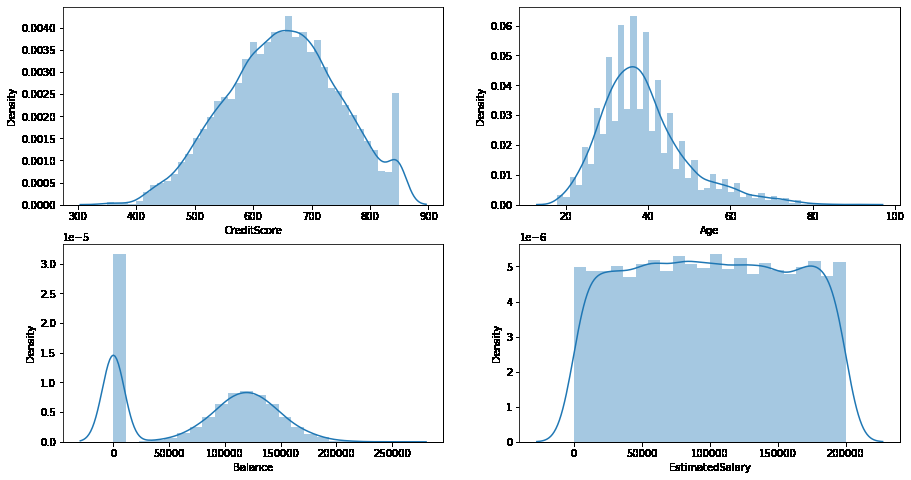We can also check the categorical count of each category in Geography and Gender.

## Python3

 `lis2 ``=` `[``'Geography'``, ``'Gender'``]``plt.subplots(figsize``=``(``10``, ``5``))``index ``=` `1`` ` `for` `col ``in` `lis2:``    ``y ``=` `dataset[col].value_counts()``    ``plt.subplot(``1``, ``2``, index)``    ``plt.xticks(rotation``=``90``)``    ``sns.barplot(x``=``list``(y.index), y``=``y)``    ``index ``+``=` `1`

Output :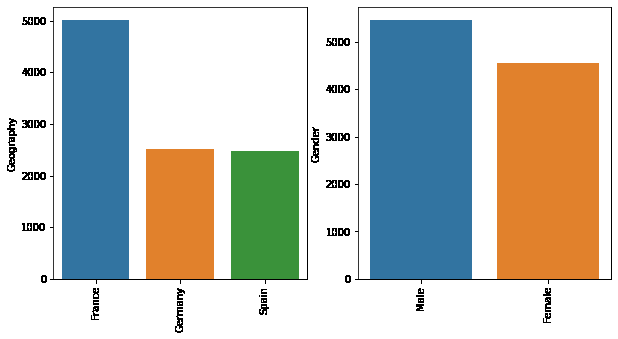## Data Preprocessing

Data preprocessing is used to convert raw data into a clear format. Raw data consist of missing values, noisy data, and raw data may be text, image, numeric values, etc.

By the above definition, we understood that transforming unstructured data into a structured form is called data preprocessing. If the unstructured data is used in machine learning models to analyze or to predict, the prediction will be false because unstructured data contains missing values and unwanted data. So for good prediction, the data need to be preprocessed.

### Finding Missing Values and Handling them

Let’s observe whether null values are present.

## Python3

 `dataset.isnull().``any``()`

Output :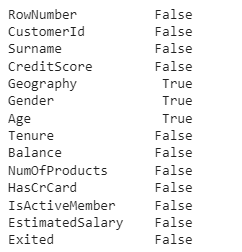Here, True indicates a null value and False indicates there is no null value. We can observe that there are 3 columns containing null values. The 3 columns are Geography, Gender, and Age. Now we need to remove the null values, to do this there are 3 ways they are:

• Deleting rows
• Replacing null with custom values
• Replacing using Mean, Median, and Mode

In this scenario, we replace null values with Mean and Mode.

## Python3

 `dataset[``"Geography"``].fillna(dataset[``"Geography"``].mode()[``0``],inplace ``=` `True``)``dataset[``"Gender"``].fillna(dataset[``"Gender"``].mode()[``0``],inplace ``=` `True``)``dataset[``"Age"``].fillna(dataset[``"Age"``].mean(),inplace ``=` `True``)`

As we know Geography and Gender is a Categorical columns we used mode and Age is an integer type so we used mean.

Note: By using “Inplace = True”, the original data set is modified.

Now once again let us check if any null values still exist.

## Python3

 `dataset.isnull().``any``()`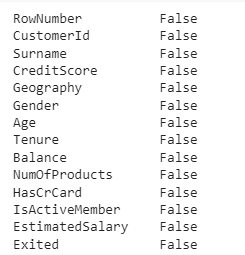### Label Encoding

Label Encoding is used to convert textual data to integer data. As we know there are two textual data type columns which are “Geography” and “Gender”.

## Python3

 `le ``=` `LabelEncoder()``dataset[``'Geography'``] ``=` `le.fit_transform(dataset[``"Geography"``])``dataset[``'Gender'``] ``=` `le.fit_transform(dataset[``"Gender"``])`

First we initialized LabelEncoder() function, then transformed textual data to integer data with fit_transform() function.

So now, the “Geography” and “Gender” columns are converted to integer data types.

### Splitting Dependent and Independent Variables

Dataset is split into x and y variables and converted to an array.

## Python3

 `x ``=` `dataset.iloc[:,``3``:``13``].values``y ``=` `dataset.iloc[:,``13``:``14``].values`

Here x is the independent variable and y is the dependent variable.

## Python3

 `x_train, x_test, y_train, y_test ``=` `train_test_split(x,y,``                                                    ``test_size ``=` `0.2``, ``                                                    ``random_state ``=` `0``)`

Here we split data into train and test sets.

### Feature Scaling

Feature Scaling is a technique done to normalize the independent variables.

## Python3

 `sc ``=` `StandardScaler()``x_train ``=` `sc.fit_transform(x_train)``x_test ``=` `sc.fit_transform(x_test)`

We have successfully preprocessed the dataset. And now we are ready to apply Machine Learning models.

## Model Training and Evaluation

As this is a Classification problem then we will be using the below models for training the data.

And for evaluation, we will be using Accuracy Score.

## Python3

 `from` `sklearn.neighbors ``import` `KNeighborsClassifier``from` `sklearn.ensemble ``import` `RandomForestClassifier``from` `sklearn.svm ``import` `SVC``from` `sklearn.linear_model ``import` `LogisticRegression`` ` `from` `sklearn ``import` `metrics`` ` `knn ``=` `KNeighborsClassifier(n_neighbors``=``3``)``rfc ``=` `RandomForestClassifier(n_estimators ``=` `7``,``                             ``criterion ``=` `'entropy'``,``                             ``random_state ``=``7``)``svc ``=` `SVC()``lc ``=` `LogisticRegression()`` ` `# making predictions on the training set``for` `clf ``in` `(rfc, knn, svc,lc):``    ``clf.fit(x_train, y_train)``    ``y_pred ``=` `clf.predict(x_test)``    ``print``(``"Accuracy score of "``,clf.__class__.__name__,``"="``,``          ``100``*``metrics.accuracy_score(y_test, y_pred))`

Output :

```Accuracy score of  RandomForestClassifier = 84.5
Accuracy score of  KNeighborsClassifier = 82.5
Accuracy score of  SVC = 86.15
Accuracy score of  LogisticRegression = 80.75```

## Conclusion

Random Forest classifier and SVC are showing the best results with an accuracy of around 85%

My Personal Notes arrow_drop_up